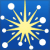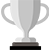## Bug in calculating cost?

Home English Bug in calculating cost?

2 voices
2 replies
• Author
Posts
•PyroDad
 Joined: Sep 2020 Posts: 11 Location: Espoo, Uusimaa, FinlandNewcomer
Likes:

Hi,

I discovered a possible bug.

I have a show where I entered only the prices of the effects in the effects file, not the cost. I wanted to add also the cost which is 25% lower. So, what I did is that I exported the table to excel, created a formula to multiply the price by .75, and re-imported the output csv into the same effects file. However, some of the effect costs had a three digit decimal part (e.g. 5.325). As the result Finale3D calculated the total cost of the show wrong. When I realized where the issue was, I was able to get around it by using excel’s TEXT() function and limiting the number of decimals. However, I consider this as a bug.DrewFinale
 Joined: Dec 2019 Posts: 370 Location: United StatesSilver
Likes:

Thanks for the bug report. I did a quick test but wasn’t able to reproduce the problem. Here are the steps I followed:

1) Create an effect with a Cost of \$5.325 and Price of \$7.10

2) Add 10 of the item to the show

3) Observe the Price total in the lower right corner of the Design window is \$7.10 x 10 = \$71.10

4) Go to ‘File > User settings’ and enable ‘Show cost instead of price in 3D view’

5) Observe the Cost total in the lower right corner of the Design window is \$5.325 x 10 = \$53.25

You didn’t mention where you are looking at the total cost. Perhaps I’m looking in a different location? If you can give me a set of steps to reproduce the issue then I would be happy to investigate further.PyroDad
 Joined: Sep 2020 Posts: 11 Location: Espoo, Uusimaa, FinlandNewcomer
Likes:

Hi Drew,

Thanks for the answer. If I remember correctly, the wrong cost was shown at the lower right corner of the main simulation window. It looked to me that the subroutine displaying the cost interpreted the dot as a thousand separator or something like that.

If you cannot replicate this issue with the info above, I’ll try to generate exact steps within the next week or so.

++karri